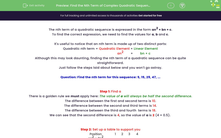# Find the Nth Term of Complex Quadratic Sequences

In this worksheet, students find expressions which represent quadratic sequences (in the form an² + bn + c) and apply these to find unknown terms.Key stage:  KS 4

Year:  GCSE

GCSE Subjects:   Maths

GCSE Boards:   AQA, Eduqas, Pearson Edexcel, OCR,

Curriculum topic:   Algebra

Curriculum subtopic:   Sequences

Difficulty level:#### Worksheet Overview

The nth term of a quadratic sequence is expressed in the form an2 + bn + c.

To find the correct expression, we need to find the values for a, b and c.

It's useful to notice that an nth term is made up of two distinct parts:

an2        +        bn + c

Although this may look daunting, finding the nth term of a quadratic sequence can be quite straightforward.

Just follow the steps laid about below and you won't go astray.

Question: Find the nth term for this sequence: 5, 15, 29, 47, ...

Step 1: Find a

There is a golden rule we must apply here: The value of a will always be half the second difference.

The difference between the first and second terms is 10.

The difference between the second and third terms is 14.

The difference between the third and fourth  terms is 18.

We can see that the second difference is 4, so the value of a is 2 (4 × 0.5).

Step 2: Set up a table to support you

 Position 1 2 3 4 an2 = 2n2 bn + c Target 5 15 29 47

In this table, the 'Position' is just the value of n (i.e. where it is in the sequence).

We have just found the value of a so we can fill in the second line by substituting values of n into 2n2:

 Position 1 2 3 4 an2 = 2n2 2 8 18 32 bn + c Target 5 15 29 47

Step 3: Find the value of bn + c

In our table, we now know the value of the quadratic element, so we can now us this to find the linear element.

These are just the differences between an2 and the target:

 Position 1 2 3 4 an2 = 2n2 2 8 18 32 bn + c 3 7 11 15 Target 5 15 29 47

We can now see that these numbers in blue (3, 7, 11, 15) form a linear sequence with an nth term of 4n - 1.

Step 4: Put it all together

Earlier, we said that...

an2        +        bn + c

2n2     +    4n - 1

There are many steps here but, if we follow them systematically, we will reach an accurate quadratic expression each time.

In this activity, we will find quadratic expressions which represent sequences and apply these to find unknown terms.

As these methods can be long and complex, it is essential to have a pen and paper handy so you can compare your working out to the given example.

### What is EdPlace?

We're your National Curriculum aligned online education content provider helping each child succeed in English, maths and science from year 1 to GCSE. With an EdPlace account you’ll be able to track and measure progress, helping each child achieve their best. We build confidence and attainment by personalising each child’s learning at a level that suits them.

Get started